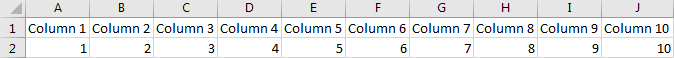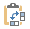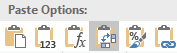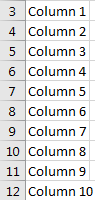Sometimes we may have so many columns in one worksheet and user cannot view all column headers in one page due to width limit. In this case user has to drag the scrollbar to check the column headers which are located in the columns invisible in current page like column U, column V etc. Is there a convenient way to check column headers directly without drag the scrollbar? This article will help you to resolve this issue by convert column headers to row headers in excel.

Prepare a table with 10 columns, and the column headers are column 1, column 2… till column 10.If you want to convert column headers into row headers by a quick way, you can use Paste Special function to implement our requirement. It can transpose a row to a column, in this case column headers saved in row1 can be transposed to specific column properly, and then user can check column headers quickly. Please follow below steps to implement it.

Step 1: Select the range you want to transpose from row to column. In this case if you want to only check the column headers in row for convenient you can only select A1 to J1.

Step 2: Click Ctrl+C to copy this row (the selected range).

Step 3: In A3 (or other cell you want to paste the column headers), right click to load menu.Step 4: In Paste Options, select Transpose (T) iconto implement transpose.Step 5: Check the result. Verify that column headers are listed in one column properly.This is just a quick way to help you to view all column headers in one column by convenient; if you want all data transpose from row to column (or column to row), you can select all data range to do transpose.

Related Posts

Calculate Number of Hours between Two Times

Calculating the difference between two times might be a valuable statistic for subsequent computations or averages, whether you're producing a time sheet for staff or recording personal exercises. While Excel has a plethora of complex functions, including date and time ...

Calculate Loan Interest in Given Year

When you borrow money, you are supposed to repay it gradually. Lenders, on the other hand, want to be compensated for their services and the risk they incur by lending you money. That is, you will not just repay the ...

Calculate Interest Rate for Loan

The interest rate is the fee charged by a lender to a borrower and is expressed as a percentage of the principal—the lent amount. The interest rate on a loan is often expressed as an annual percentage rate, abbreviated as ...

Calculate Interest for Given Period

Using the IPMT function in Excel, we can compute the interest payment on any loan. This step-by-step tutorial will guide Excel users of all skill levels through the process to calculate interest for given period. Finally, the formula: =IPMT(B3/12,1,B5,-B2) The ...

How To Use Excel GCD Function

This post will guide you how to use Excel GCD function with syntax and examples in Microsoft excel. Description The Excel GCD function Returns the greatest common divisor of two or more integers. So you can use the GCD function ...

Calculate A Ratio From Two Numbers In Excel

In elementary mathematics, a ratio is a connection or comparison between two or more integers. For example, ratios are often expressed as ":" to demonstrate the relationship between two numbers. You would think that manually calculating a ratio from two ...

How To Use Excel RRI Function

This post will guide you how to use Excel RRI function with syntax and examples in Microsoft excel. Description The Excel RRI function Returns an equivalent interest rate for the growth of an investment. So you can use the RRI ...

CAGR Formula Examples in Excel

CAGR in Excel is a formula that calculates the compound annual growth rate for any invested amount over the specified years or timeframe. Although there is no direct function in Excel that can help us identify the CAGR value, there ...

Build Hyperlink With VLOOKUP in Excel

You might have come across a task in which you were assigned to build hyperlinks, which seems very easy, and if you are new to excel or don't have enough experience with it, then you might wonder about doing this ...

Break ties with helper COUNTIF and column

Suppose you got a task to adjust the values that contain the ties; what would be your first attempt to break the ties of the given value? If you are wondering about doing this task manually, let me add that ...

Sidebar